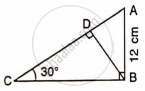# Find: Bc - Mathematics

Sum

Find: BC#### Solution

From right triangle ABC

tan 30° = "AB"/"BC"

(1)/sqrt(3) = (12)/"BC"

BC = 12sqrt(3) "cm".

Concept: Solution of Right Triangles
Is there an error in this question or solution?

#### APPEARS IN

Selina Concise Mathematics Class 9 ICSE
Chapter 24 Solution of Right Triangles [Simple 2-D Problems Involving One Right-angled Triangle]
Exercise 24 | Q 13.1 | Page 304